Reliability and Survival Methods > Survival Analysis > Statistical Reports for Survival Analysis
Publication date: 05/24/2021

# Statistical Reports for Survival Analysis

For data that is not interval censored, the initial reports show Summary and Quantiles data (Figure 13.15). The Summary data shows the number of failed and number of censored observations for each group (when there are groups) and for the whole study. The mean and standard deviations are also adjusted for censoring. For computational details about these statistics, see the LIFETEST Procedure chapter in SAS Institute Inc. (2020).

The Quantiles data shows time to failure statistics for individual and combined groups. These include the median survival time, with upper and lower 95% confidence limits. The median survival time is the time (number of days) at which half the subjects have failed. The quartile survival times (25% and 75%) are also included.

Figure 13.15 Summary Statistics for the Univariate Survival Analysis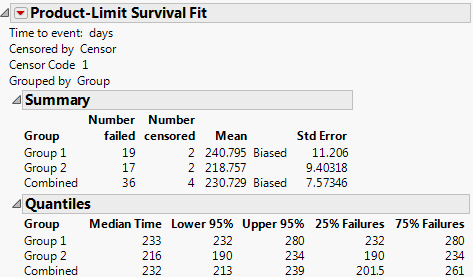The Summary report gives estimates for the mean survival time, as well as the standard error of the mean. The estimated mean survival time is defined as follows: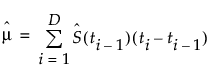with a standard error of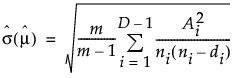where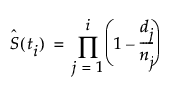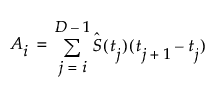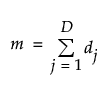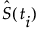is the survival distribution at time ti

D is the number of distinct event times

ni is the number of surviving units just prior to ti

di is the number of units that fail at ti

t0 is defined to be 0

When there are multiple groups, the Tests Between Groups table provides statistical tests for homogeneity among the groups. Kalbfleisch and Prentice (1980, ch. 1), Hosmer and Lemeshow (1999, ch. 2), and Klein and Moeschberger (1997, ch. 7) discuss statistics and comparisons of survival curves.

Figure 13.16 Tests between Groups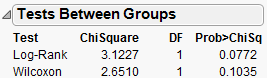Test

Names two statistical tests of the hypothesis that the survival functions are the same across groups.

Chi-Square

Provides the Chi-square approximations for the statistical tests.

The Log-Rank test places more weight on larger survival times and is more useful when the ratio of hazard functions in the groups being compared is approximately constant. The hazard function is the instantaneous failure rate at a given time. It is also called the mortality rate or force of mortality.

The Wilcoxon test places more weight on early survival times and is the optimum rank test if the error distribution is logistic. See Kalbfleisch and Prentice (1980).

DF

Provides the degrees of freedom for the statistical tests.

Prob>ChiSq

Lists the probability of obtaining a Chi-square value greater than the one computed if the survival functions are the same for all groups.

Figure 13.17 shows an example of the product-limit survival function estimates for one group.

Figure 13.17 Example of Survival Estimates Table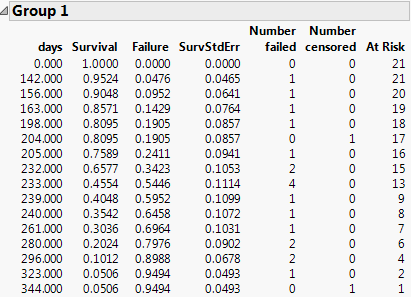Note: When the final time recorded is a censored observation, the report indicates a biased mean estimate. The biased mean estimate is a lower bound for the true mean.